# 2021 A-level H1 Mathematics (8865) Paper 1 Suggested SolutionsAll solutions here are suggested. Mr. Teng will hold no liability for any errors. Comments are entirely personal opinions. I will not be doing much formatting in this post.

Q1 [Maximum marks: 4]

Discriminant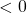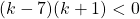Q2 [Maximum marks: 5]

(a)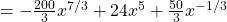(b)Q3

Q4

Q5

Q6 [Maximum marks: 4]
Let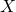be the height of a sunflower plant, in m.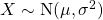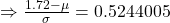— (1)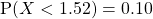— (2)
Using GC,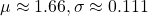Q7 [Maximum marks: 4]
Observe that all the letters are distinct. Aside from P and G, we need toletters to put them between P and G.
Number of ways to form the group “P X X X X X G” with arrangements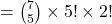We then need to arrange the group formed with the remaining 2 letters.
Total number of waysQ8 [Maximum marks: 8]
(a)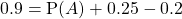(b) It is the probability that both events A and B do not occur.
Observe by drawing a venn diagram that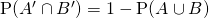.(c)Since, events A and B are not independent.
(d)

Q9 [Maximum marks ] – CLT Topic

Q10 [Maximum marks: 11]

(a)

(b)
Required probability(c)
Required probability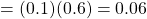(d)
Required probability11 [Maximum marks: 11]

(a) Letbe the number of orders delivered within 24 hours, out of 10 orders.(i) Required probability(ii) Required probability(b)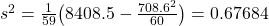Letbe population mean delivery time.
Testagainstat 5% significance level.
Under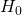,approximately by central limit theorem.
From GC,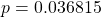Since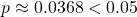, we rejectand conclude that there is sufficient evidence at 5% significance level that the mean delivery time is less than 12 hours. Thus, manager’s claim is supported by the data.

(c) Since the sample sizeis sufficiently large for the sample mean distribution of delivery times to be approximated to a normal distribution by central limit theorem, it is not necessary to assume the distribution of the deliver times.

12 [Maximum marks: 13]

(a)(b)Let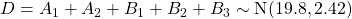(c)
Letbe the interval timings, in minutes.Let.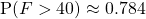(d)
Letbe the second half duration, in minutes.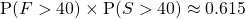.

(e)
The events found in (d) is a subset of the events found in that of “the two halves of the concert last for a total of more than 80 minutes”.

Not readable? Change text.